# A preview of new features in bootnet 1.1

This blog post was written by Sacha Epskamp.

In the past year, we have published two tutorial papers on the bootnet package for R, which aims to provide an encompassing framework for estimating network structures and checking their accuracy and stability. The first paper, published in Behavior Research Methods, focuses on how to use the bootstrapping methods to assess the accuracy and stability of network structures. The second, published in Psychological Methods, focuses on the most commonly used network model, the Gaussian graphical model (GGM; a network of partial correlations), and discusses further utility of the bootnet package.

With more than a year of development, version 1.1 of the bootnet package marks the most ambitious series of updates to the package to date. The version is now ready for testing on Github, and will soon be released on CRAN. This version includes or fleshes out a total of 7 new default sets, including one default set aimed at time-series analysis, and offers new functionality to the default sets already included in the package. In addition, the package now fully supports directed networks as well as methods resulting in multiple network models, and supports the expected influence centrality measure. Furthermore, the plotting functions in bootnet have been updated and now show more information. Finally, the package includes a new simulation function, replicationSimulator, and greatly improves the functionality of the netSimulator function. With these extensions, bootnet now aims to provide an exhaustive simulation suite for network models in many conditions. This blog post is intended to introduce this new functionality and to encourage testing of the functionality before the package is submitted to CRAN.

The package can be installed using:

library("devtools")
install_github("sachaepskamp/bootnet")


which requires developer tools to be installed: Rtools for Windows and Xcode for Mac. Subsequently, the package can be loaded as usual:
The package can be installed using:

library("bootnet")


Bootnet now contains the following default sets:

default model Description Packages Recently added
“EBICglasso” GGM Regularized GGM estimation using glasso and EBIC Model selection qgraph, glasso
“pcor” GGM Unregularized GGM estimation, possibly thresholded using significance testing or FDR qgraph
“IsingFit” Ising Regularized Ising models using nodewise logistic regressions and EBIC model selection IsingFit, glmnet
“IsingSampler” Ising Unregularized Ising moddel estimation IsingSampler
“huge” GGM Regularized GGM estimation using glasso and EBIC Model selection huge
“mgm” Mixed graphical model Regularized mixed graphical model estimation using EBIC or cross-validation mgm Revamped
“relimp” Relative importance networks Relative importance networks, possible using another default for network structure relaimpo Yes
“cor” Correlation networks Correlation networks, possibly thresholded using significance testing or FDR qgraph Yes
“TMFG” Correlation networks & GGM Triangulated Maximally Filtered Graph NetworkToolbox Yes
“LoGo” GGM Local/Global Sparse Inverse Covariance Matrix NetworkToolbox Yes
“ggmModSelect” GGM Unregularized (E) BIC model selection using glasso and stepwise estimation qgraph, glasso Yes
“graphicalVAR” Graphical VAR Regularized estimation of temporal and contemporaneous networks graphicalVAR Yes

As can be seen, several of these are newly added. For example, we can now estimate the two recently added more conservative GGM estimation methods added to qgraph, which I described in an earlier blog post. Taking my favorite dataset as example:

library("psych")
data(bfi)


The optimal unregularized network that minimizes BIC (note that tuning defaults to 0 in this case) can be obtained as follows:

net_modSelect <- estimateNetwork(bfi[,1:25],
default = "ggmModSelect",
stepwise = FALSE,
corMethod = "cor")


It is generally recommended to use the stepwise improvement of edges with stepwise = TRUE, but I set it to FALSE here to make this tutorial easier to follow. Likewise, the thresholded regularized GGM can be obtained using the new threshold argument for the EBICglasso default set:

net_thresh <- estimateNetwork(bfi[,1:25],
tuning = 0, # EBICglasso sets tuning to 0.5 by default
default = "EBICglasso",
threshold = TRUE,
corMethod = "cor")


As typical, we can plot the network:

Layout <- qgraph::averageLayout(net_modSelect, net_thresh)
layout(t(1:2))
plot(net_modSelect, layout = Layout, title = "ggmModSelect")
plot(net_thresh, layout = Layout, title = "Thresholded EBICglasso")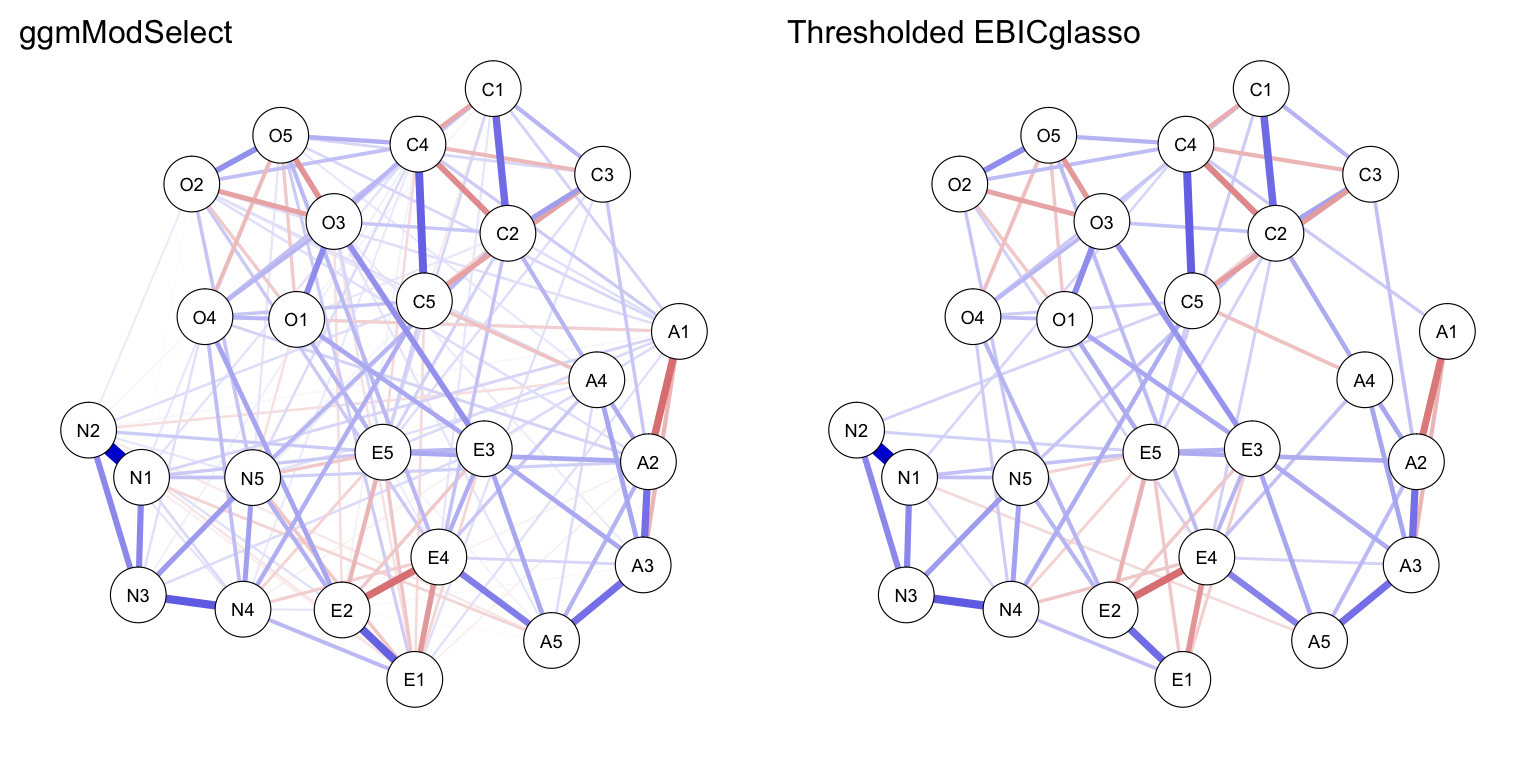## Principal direction and expected influence

One robust finding in psychological literature is that all variables tend to correlate positively after arbitrary rescaling of variables – the positive manifold. Likewise, it is common to expect parameters focusing on conditional associations (e.g., partial correlations) to also be positive after rescaling variables. Negative edges in such a network could indicate (a) violations of the latent variable model and (b) the presence of common cause effects in the data. To this end, bootnet now includes the ‘principalDirection’ argument in many default sets, which takes the first eigenvector of the correlation matrix and multiplies all variables by their corresponding sign. For example:

net_modSelect_rescale <- estimateNetwork(bfi[,1:25],
default = "ggmModSelect",
stepwise = FALSE,
principalDirection = TRUE)

net_thresh_rescale <- estimateNetwork(bfi[,1:25],
tuning = 0,
default = "EBICglasso",
threshold = TRUE,
principalDirection = TRUE)

layout(t(1:2))
plot(net_modSelect_rescale, layout = Layout,
title = "ggmModSelect")
plot(net_thresh_rescale, layout = Layout,
title = "Thresholded EBICglasso")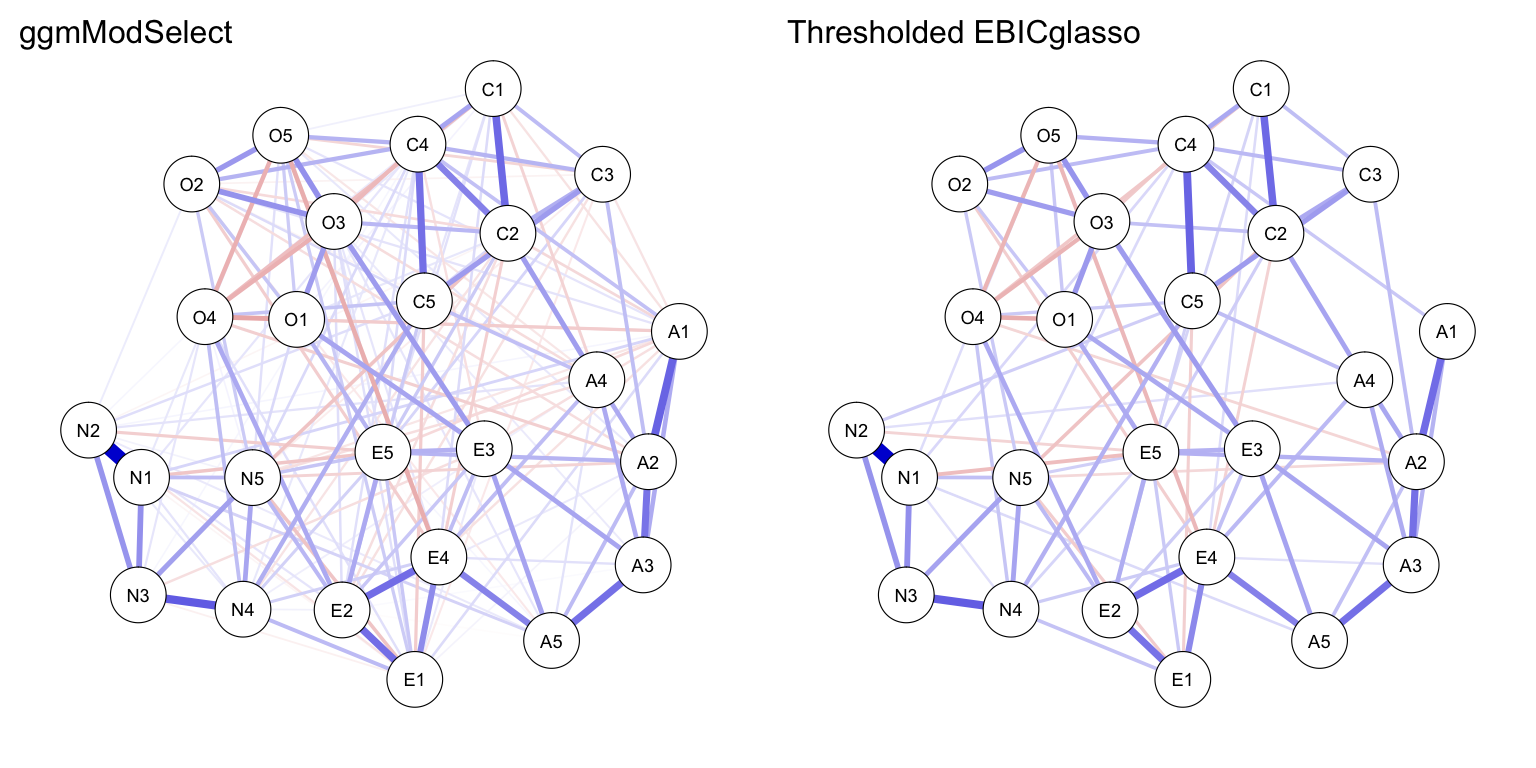This makes the edges of an unexpected sign much more profound, at the cost of interpretability (as now the rescaling of some variables has to be taken into account).

One potentially useful centrality index that can be used on graphs mostly showing only positive relationships (and all variables recoded in the same direction) is the newly proposed expected influence measure. Expected influence computes node strength without taking the absolute value of edge-weights. This centrality measure can now be obtained:

qgraph::centralityPlot(
list(
ggmModSelect = net_modSelect_rescale,
EBICGlasso_thresh = net_thresh_rescale
), include = "ExpectedInfluence"
)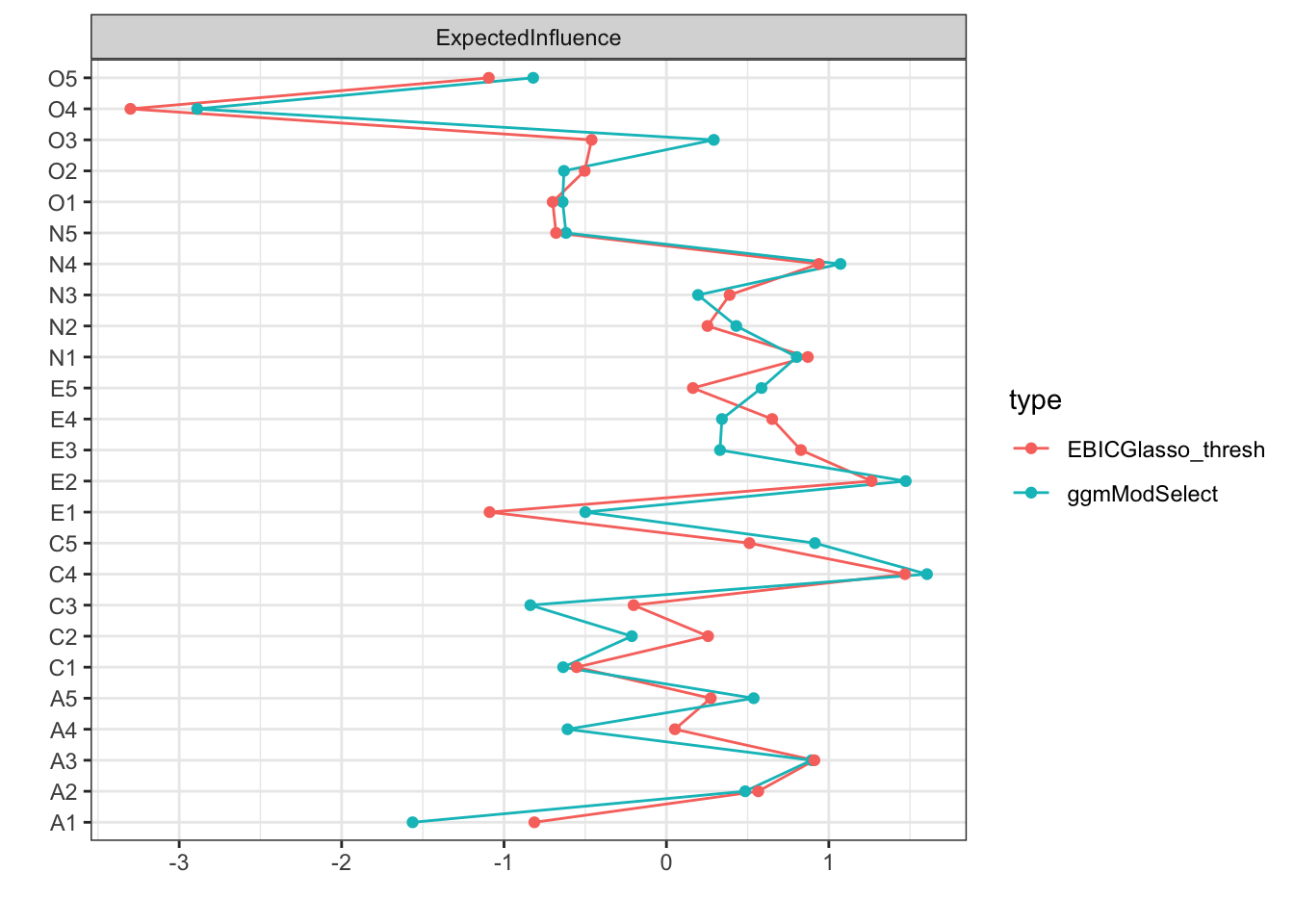To bootstrap expected influence, it has to be requested from bootnet():

boots <- bootnet(net_thresh_rescale, statistics = "ExpectedInfluence",
nBoots = 1000, nCores = 8, type = "case")

library("ggplot2")
plot(boots, statistics = "ExpectedInfluence") +
theme(legend.position = "none")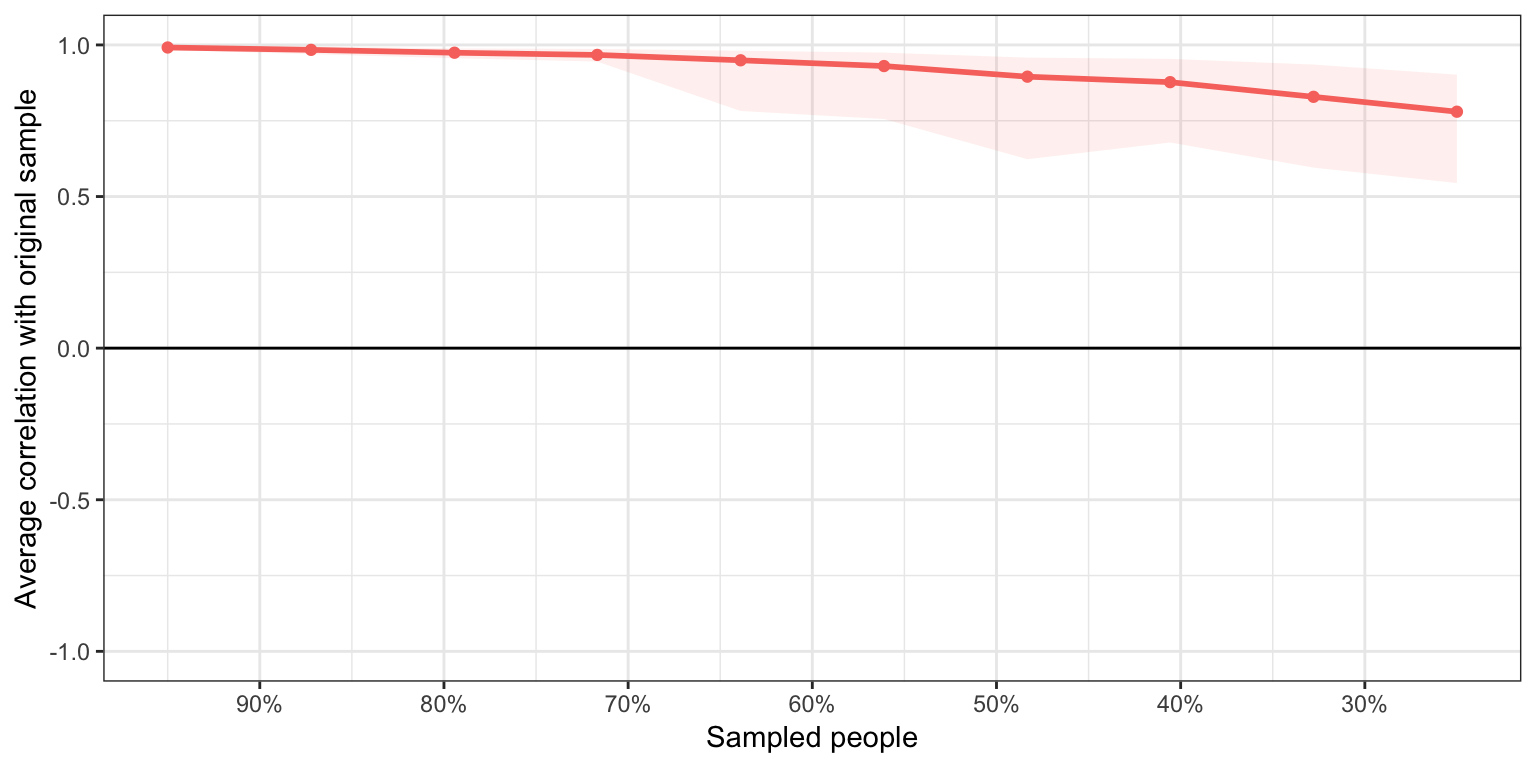In addition to expected influence, thanks to the contribution of Alex Christensen, randomized shortest paths betweenness centrality (RSPBC) and hybrid centrality are now also supported, which can be called using statistics = c("rspbc", "hybrid").

## Relative importance networks

Relative importance networks can be seen as a re-parameterization of the GGM in which directed edges are used to quantify the (relative) contribution in predictability of each variable on other variables. This can be useful mainly for two reasons: (1) the relative importance measures are very stable, and (2) centrality indices based on relative importance networks have more natural interpretations. For example, in-strength of non-normalized relative importance networks equals $$R^2$$. Bootnet has been updated to support directed networks, which allows for support for estimating relative importance networks. The estimation may be slow with over 20 variables though. Using only the first 10 variables of the BFI dataset we can compute the relative importance network as follows:

net_relimp <- estimateNetwork(bfi[,1:10],
default = "relimp",
normalize = FALSE)


As the relative importance network can be seen as a re-parameterization of the GGM, it makes sense to first estimate a GGM structure and subsequently impose that structure on the relative importance network estimation. This can be done using the structureDefault argument:

net_relimp2 <- estimateNetwork(bfi[,1:10],
default = "relimp",
normalize = FALSE,
structureDefault = "ggmModSelect",
stepwise = FALSE # Sent to structureDefault function
)

Layout <- qgraph::averageLayout(net_relimp, net_relimp2)
layout(t(1:2))
plot(net_relimp, layout = Layout, title = "Saturated")
plot(net_relimp2, layout = Layout, title = "Non-saturated")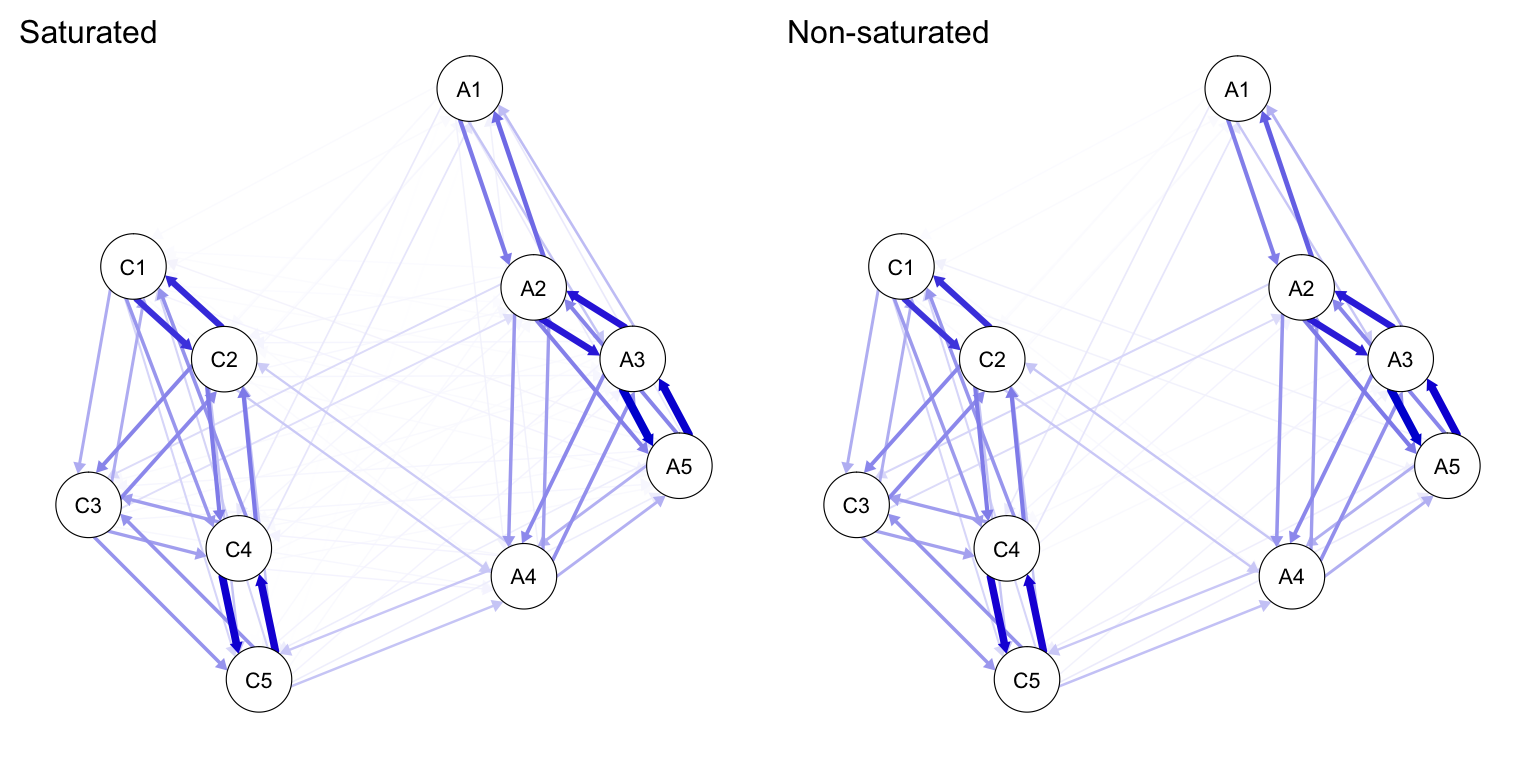The difference is hardly visible because edges that are removed in the GGM structure estimation are not likely to contribute a lot of predictive power.

## Time-series analysis

The LASSO regularized estimation of graphical vector auto-regression (VAR) models, as implemented in the graphicalVAR package, is now supported in bootnet! For example, we can use the data and codes supplied in the supplementary materials of our recent publication in Clinical Psychological Science to obtain the detrended data object Data. Now we can run the graphical VAR model (I use nLambda = 8 here to speed up computation, but higher values are recommended and are used in the paper):

# Variables to include:
"bodily.discomfort")

# Estimate model:
gvar <- estimateNetwork(
Data, default = "graphicalVAR", vars = Vars,
tuning = 0, dayvar = "date", nLambda = 8
)


We can now plot both networks:

Layout <- qgraph::averageLayout(gvar$graph$temporal,
gvar$graph$contemporaneous)
layout(t(1:2))
plot(gvar, graph = "temporal", layout = Layout,
title = "Temporal")
plot(gvar, graph = "contemporaneous", layout = Layout,
title = "Contemporaneous")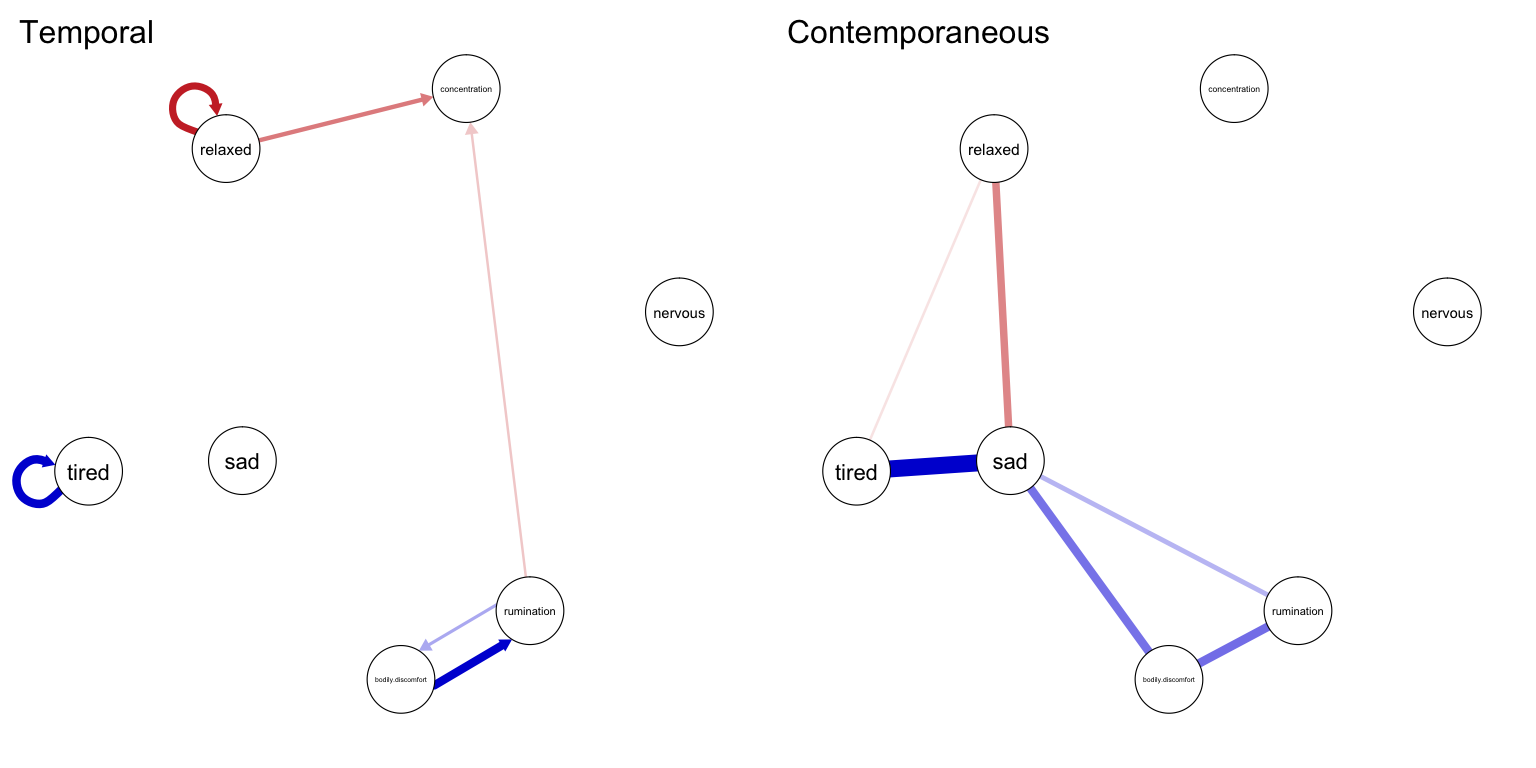The bootstrap can be performed as usual:

gvar_boot <- bootnet(gvar, nBoots = 100, nCores = 8)


To plot the results, we need to make use of the graph argument:

plot(gvar_boot, graph = "contemporaneous", plot = "interval")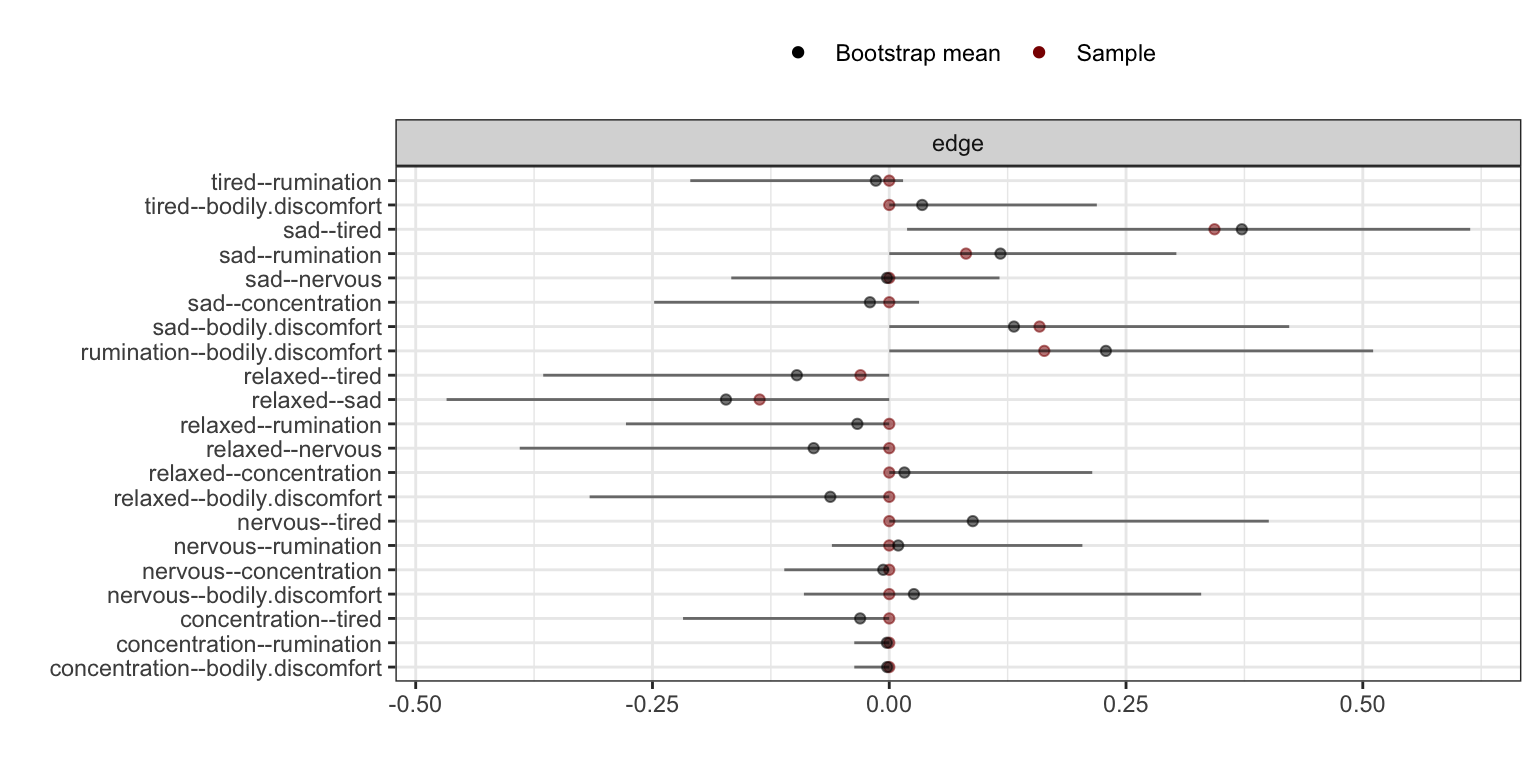## Splitting edge accuracy and model inclusion

Some of the new default sets (ggmModSelect, TMFG and LoGo) do not rely on regularization techniques to pull estimates to zero. Rather, they first select a set of edges to include, then estimate a parameter value only for the included edges. The default plotting method of edge accuracy, which has been updated to include the means of the bootstraps, will then not accurately reflect the range of parameter values. Making use of arguments plot = "interval" and split0 = TRUE, we can plot quantile intervals only for the times the parameter was not set to zero, in addition to a box indicating how often the parameter was set to zero:

# Agreeableness items only to speed things up:
net_modSelect_A <- estimateNetwork(bfi[,1:5],
default = "ggmModSelect",
stepwise = TRUE,
corMethod = "cor")

# Bootstrap:
boot_modSelect <- bootnet(net_modSelect_A, nBoots = 100, nCores = 8)

# Plot results:
plot(boot_modSelect, plot = "interval", split0 = TRUE)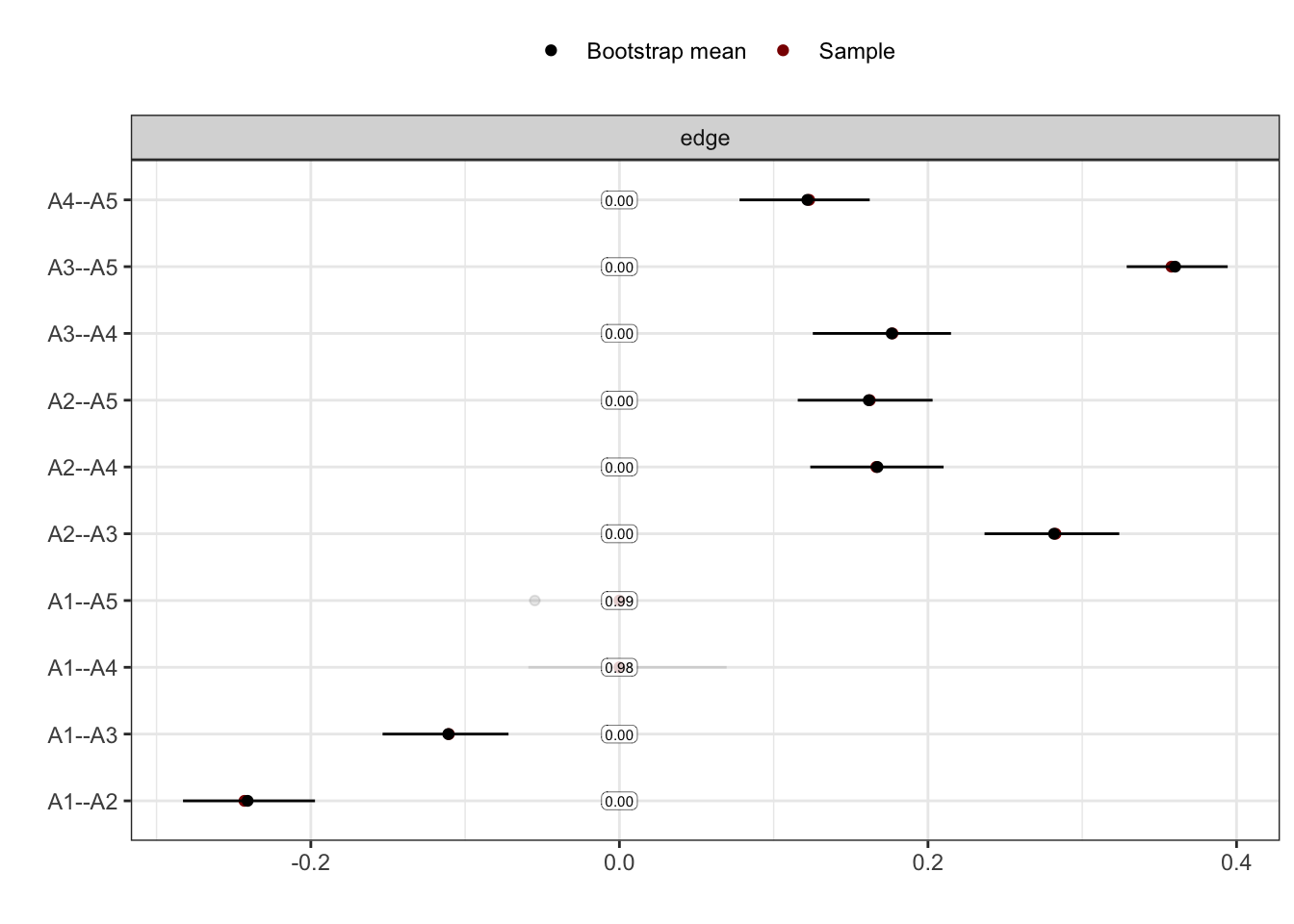This shows that the edge A1 (Am indifferent to the feelings of others) – A5 (Make people feel at ease) was always removed from the network, contradicting the factor model. The transparency of the intervals shows also how often an edge was included. For example, the edge A1 – A4 was almost never included, but when it was included it was estimated to be negative.

## Accuracy of directed networks

Accuracy plots of directed networks are now supported:

net_relimp_A <- estimateNetwork(bfi[,1:5],
default = "relimp",
normalize = FALSE)
boot_relimp_A <- bootnet(net_relimp_A, nBoots = 100, nCores = 8)
plot(boot_relimp_A, order = "sample")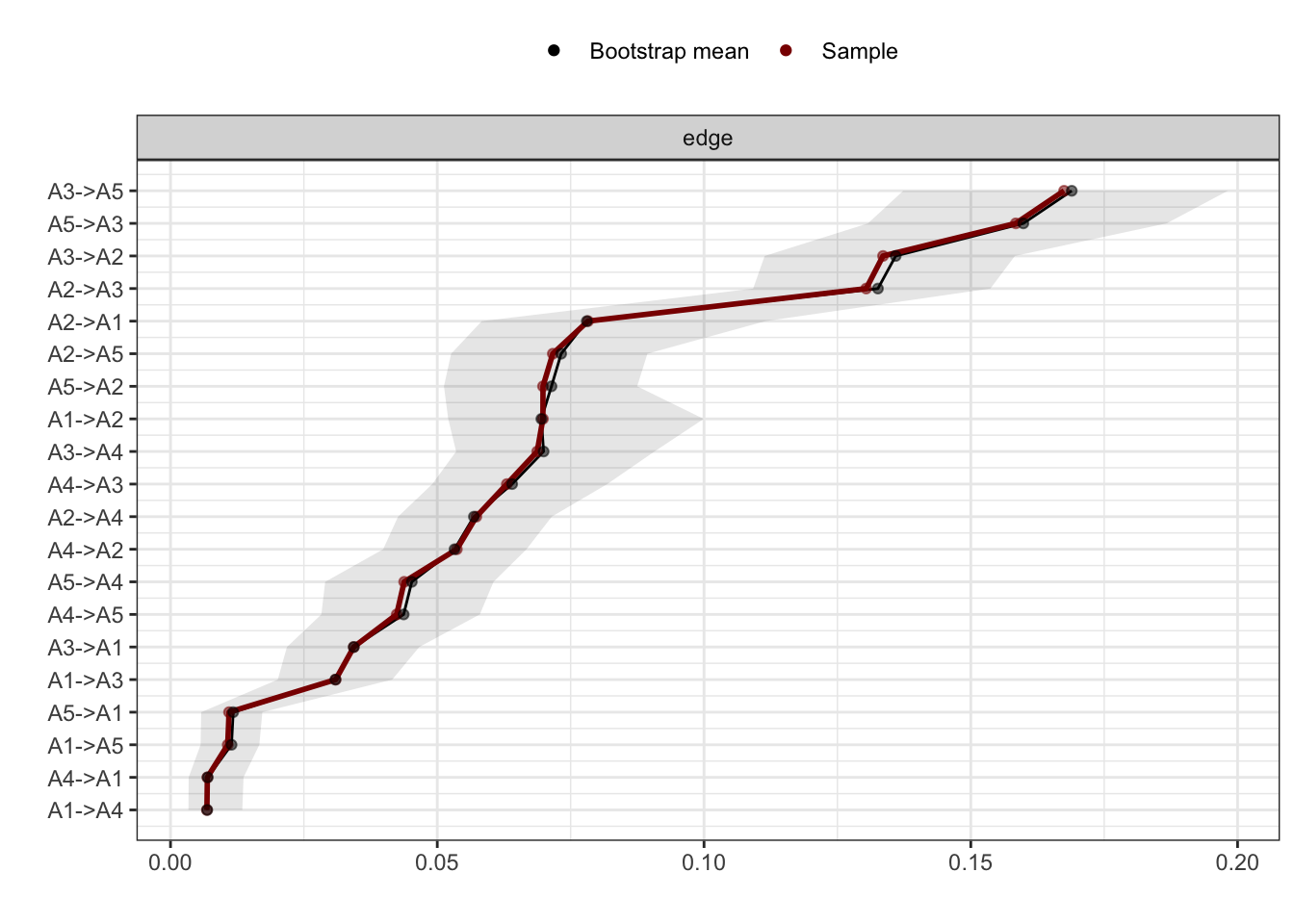# Simulation suite

The netSimulator function and accompanying plot method have been greatly expanded. The most basic use of the function is to simulate the performance of an estimation method given some network structure:

Sim1 <- netSimulator(
input = net_modSelect,
dataGenerator = ggmGenerator(),
nCases = c(100,250,500,1000),
nCores = 8,
nReps = 100,
default = "ggmModSelect",
stepwise = FALSE)

plot(Sim1)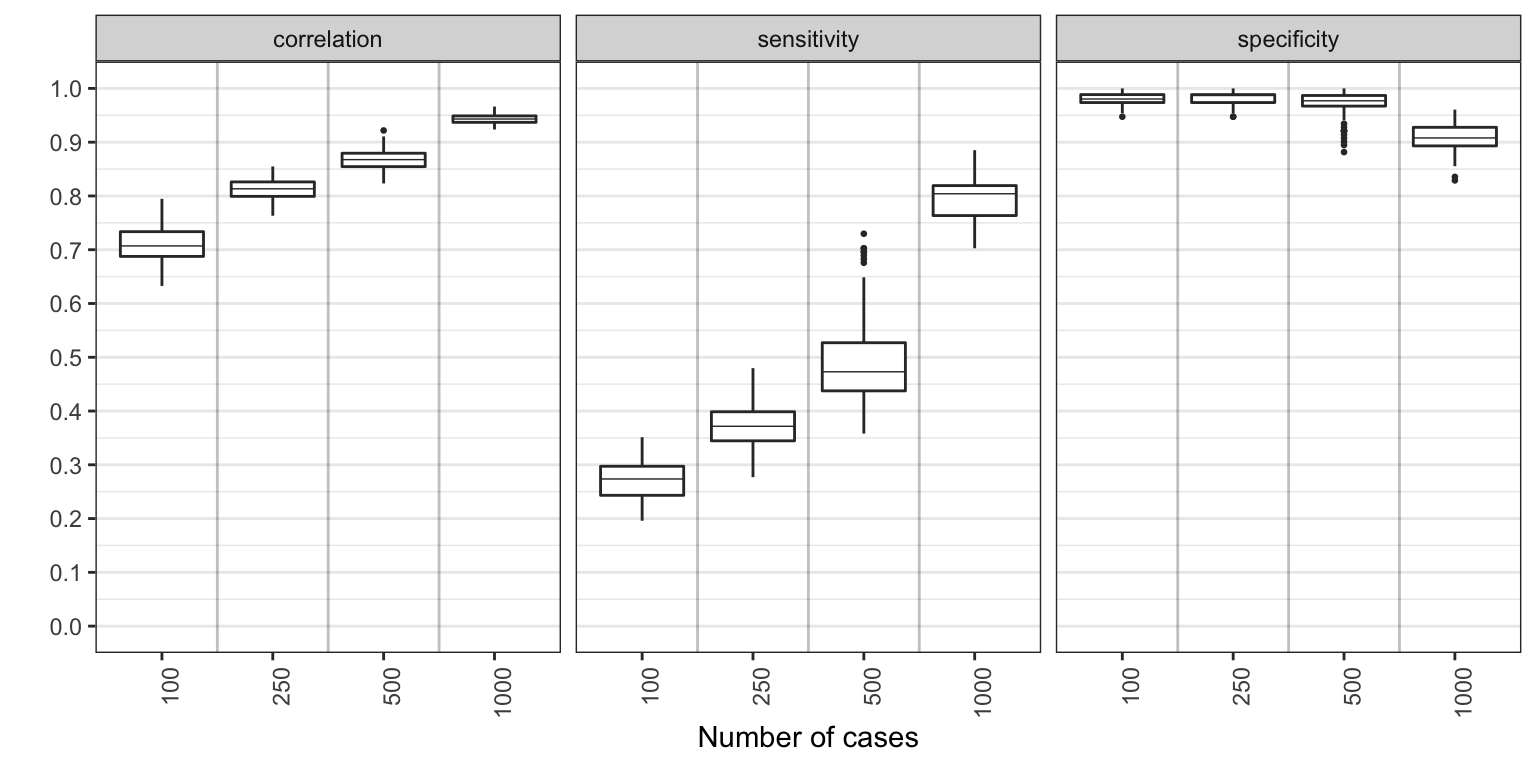Instead of keeping the network structure fixed, we could also use a function as input argument to generate a different structure every time. The updated genGGM function allows for many such structures (thanks to Mark Brandt!). In addition, we can supply multiple arguments to any estimation argument used to test multiple conditions. For example, perhaps we are interested in investigating if the stepwise model improvement is really needed in 10-node random networks with $$25\%$$ sparsity:

Sim2 <- netSimulator(
input = function()bootnet::genGGM(10, p = 0.25, graph = "random"),
dataGenerator = ggmGenerator(),
nCases = c(100,250,500,1000),
nCores = 8,
nReps = 100,
default = "ggmModSelect",
stepwise = c(FALSE, TRUE))

plot(Sim2, color = "stepwise")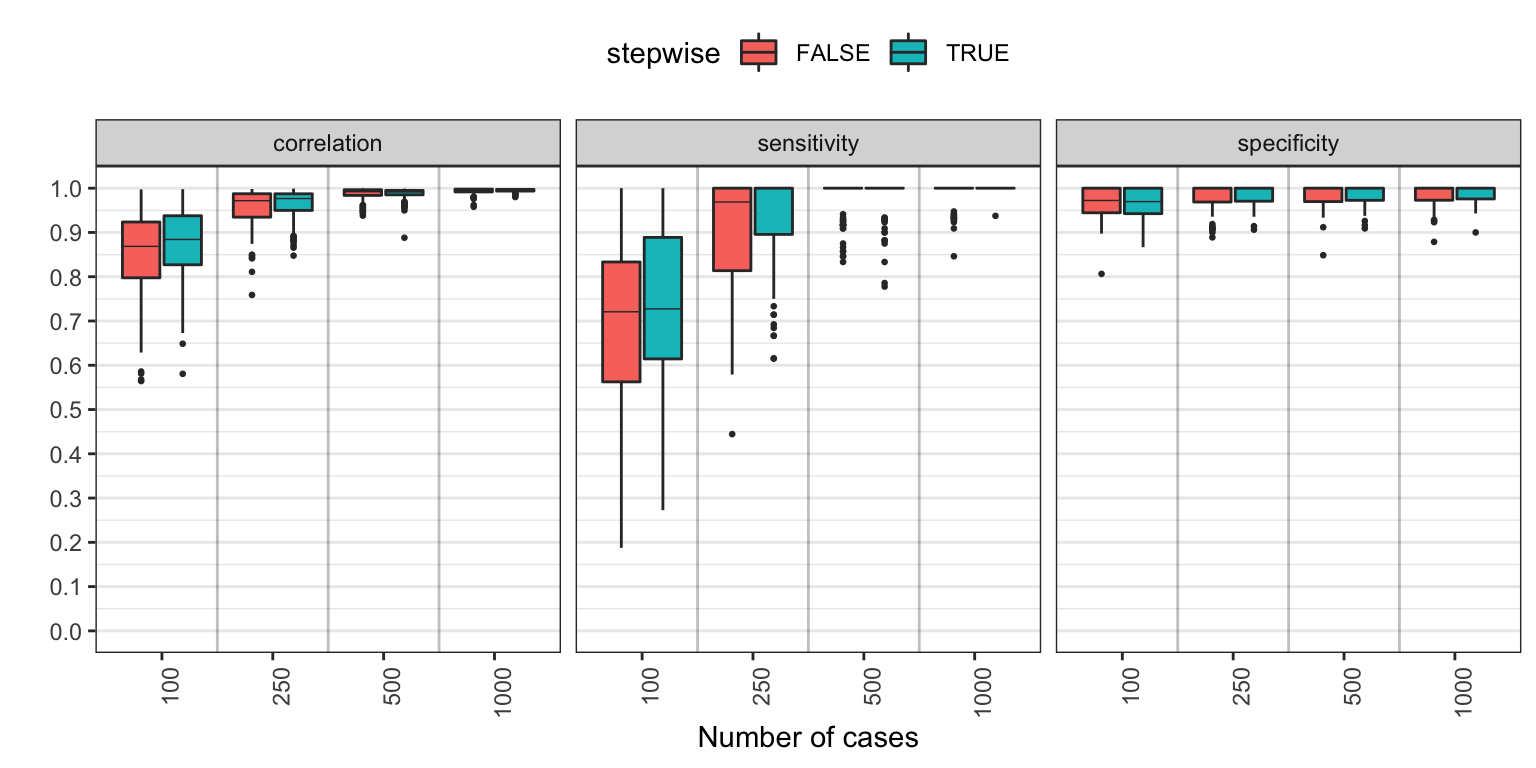which shows slightly better specificity at higher sample sizes. We might wish to repeat this simulation study for 50% sparse networks:

Sim3 <- netSimulator(
input = function()bootnet::genGGM(10, p = 0.5, graph = "random"),
dataGenerator = ggmGenerator(),
nCases = c(100,250,500,1000),
nCores = 8,
nReps = 100,
default = "ggmModSelect",
stepwise = c(FALSE, TRUE))

plot(Sim3, color = "stepwise")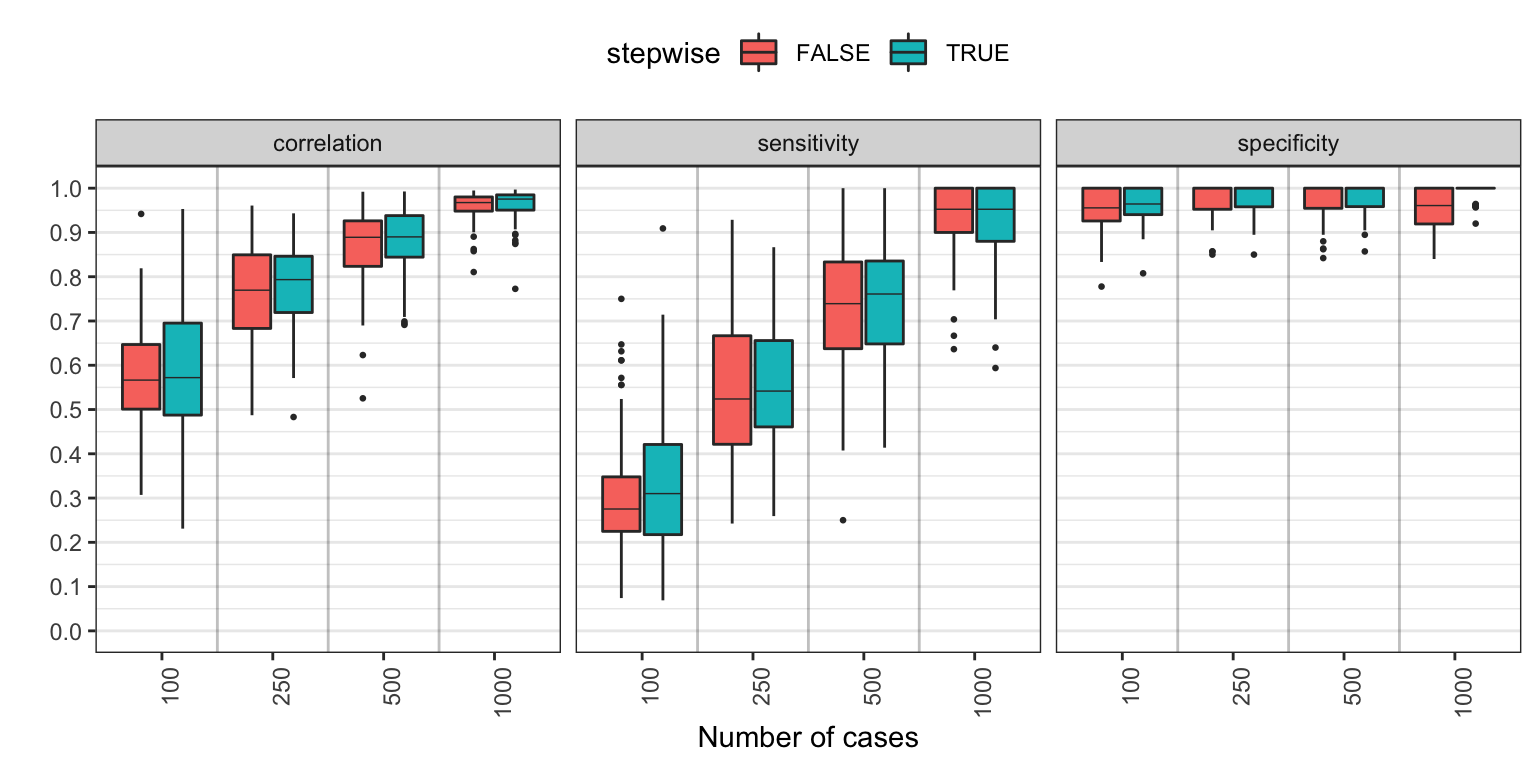The results object is simply a data frame, meaning we can combine our results easily and use the plot method very flexibly:

Sim2$sparsity <- "sparsity: 0.75" Sim3$sparsity <- "sparsity: 0.50"
Sim23 <- rbind(Sim2,Sim3)
plot(Sim23, color = "stepwise", yfacet = "sparsity")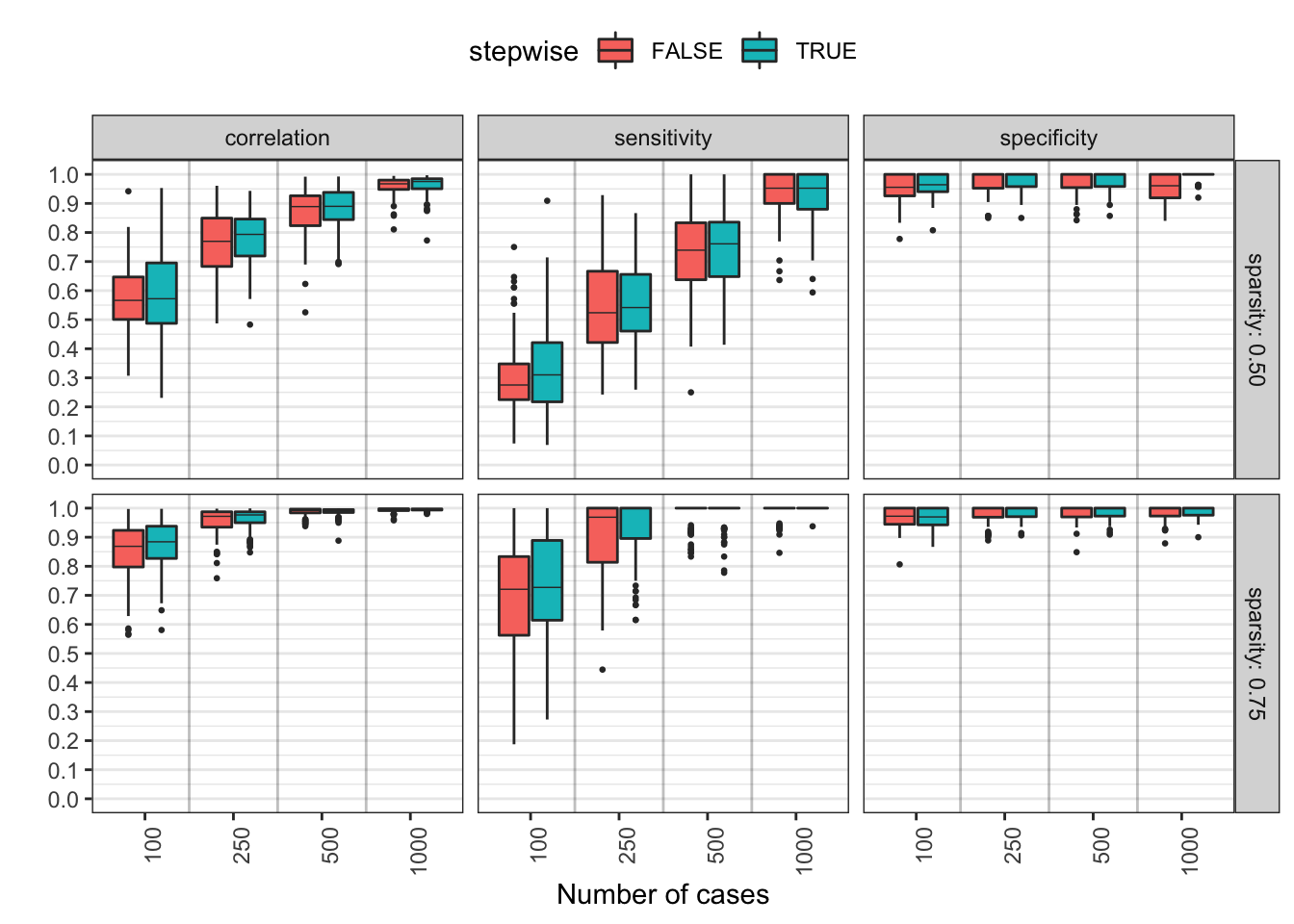Investigating the recovery of centrality (correlation with true centrality) is also possible:

plot(Sim23, color = "stepwise", yfacet = "sparsity",
yvar = c("strength", "closeness", "betweenness", "ExpectedInfluence"))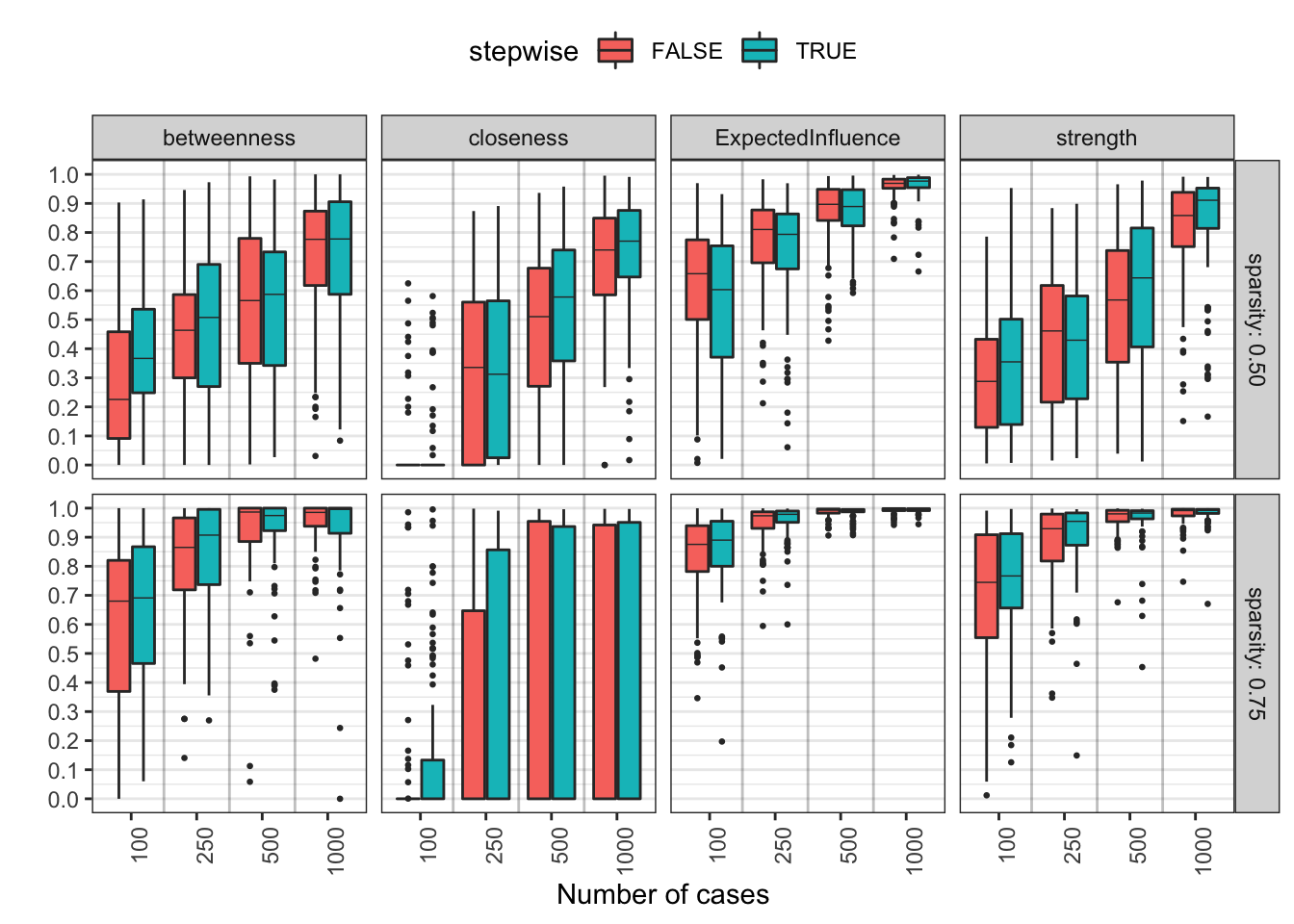Likewise, we can also change the x-axis variable:

Sim23$sparsity2 <- gsub("sparsity: ", "", Sim23$sparsity)
plot(Sim23, color = "stepwise", yfacet = "nCases",
xvar = "sparsity2", xlab = "Sparsity")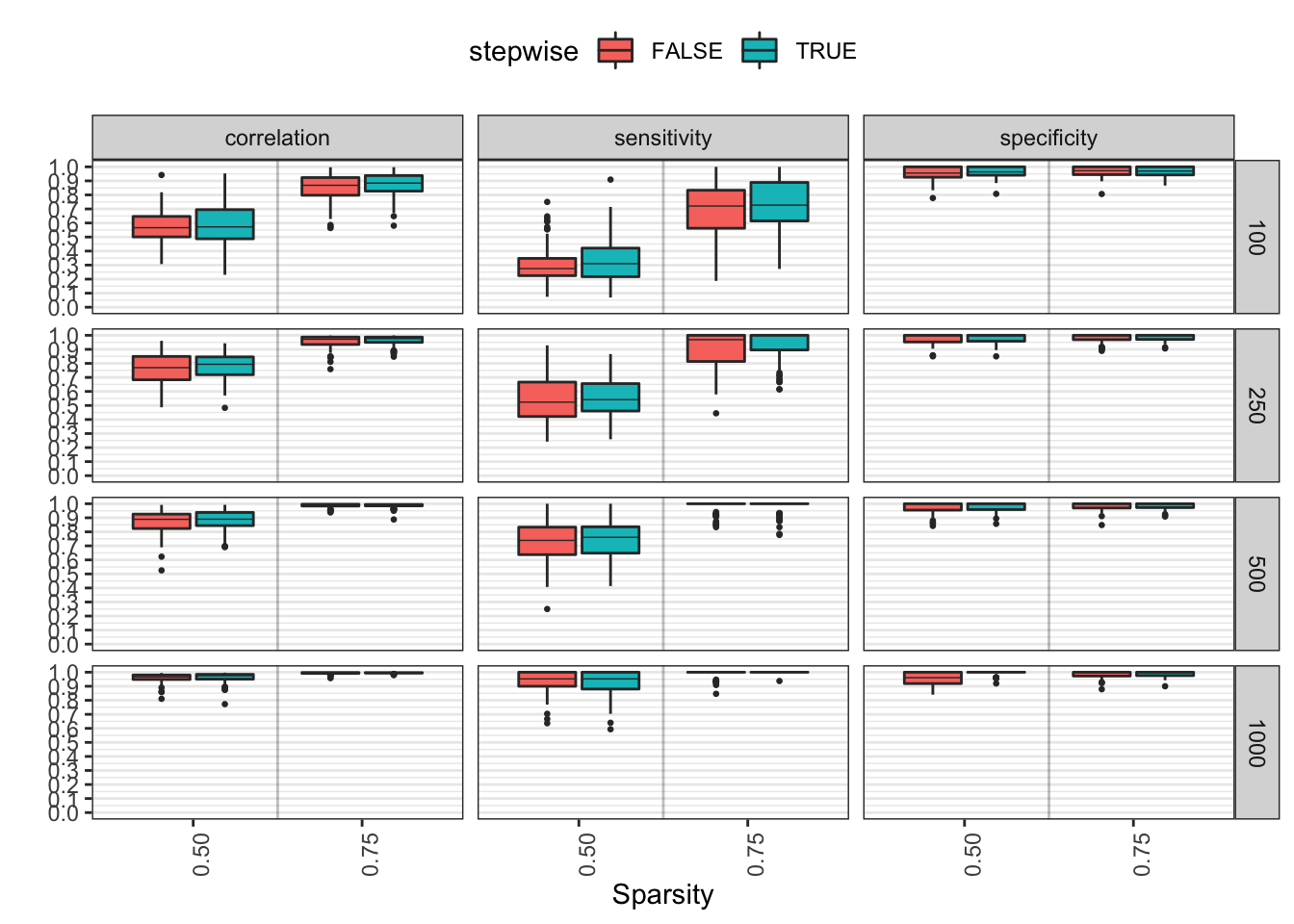This allows for setting up powerful simulation studies with minimal effort. For example, inspired by the work of Williams and Rast, we could compare such an un-regularized method with a regularized method and significance thresholding:

Sim2b <- netSimulator(
input = function()bootnet::genGGM(10, p = 0.25, graph = "random"),
dataGenerator = ggmGenerator(),
nCases = c(100,250,500,1000),
nCores = 8,
nReps = 100,
default = "EBICglasso",
threshold = c(FALSE, TRUE))

Sim2c <- netSimulator(
input = function()bootnet::genGGM(10, p = 0.25, graph = "random"),
dataGenerator = ggmGenerator(),
nCases = c(100,250,500,1000),
nCores = 8,
nReps = 100,
default = "pcor",
threshold = "sig",
alpha = c(0.01, 0.05))

Sim2$estimator <- paste0("ggmModSelect", ifelse(Sim2$stepwise,"+step","-step"))
Sim2b$estimator <- paste0("EBICglasso", ifelse(Sim2b$threshold,"+threshold","-threshold"))
Sim2b$threshold <- NULL Sim2c$estimator <- paste0("pcor (a = ",Sim2c\$alpha,")")

# Combine:
Sim2full <- dplyr::bind_rows(Sim2, Sim2b, Sim2c)

# Plot:
plot(Sim2full, color = "estimator")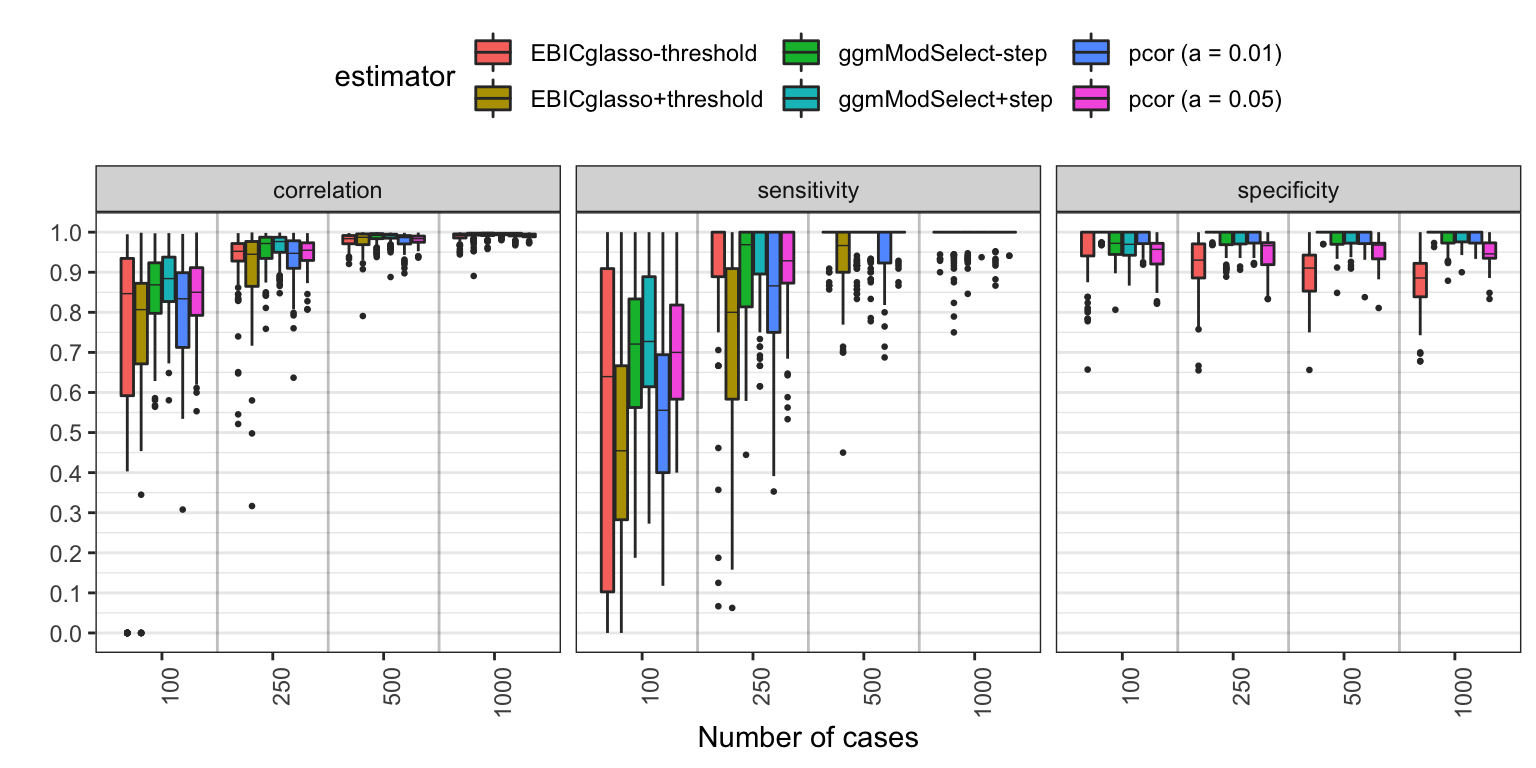## Replication simulator

In response to a number of studies aiming to investigate the replicability of network models, I have implemented a function derived from netSimulator that instead simulates two datasets from a network model, treating the second as a replication dataset. This allows researchers to investigate what to expect when aiming to replicate effect. The input and usage is virtually identical to that of netSimulator:

SimRep <- replicationSimulator(
input = function()bootnet::genGGM(10, p = 0.25, graph = "random"),
dataGenerator = ggmGenerator(),
nCases = c(100,250,500,1000),
nCores = 8,
nReps = 100,
default = "ggmModSelect",
stepwise = c(FALSE, TRUE))

plot(SimRep, color = "stepwise")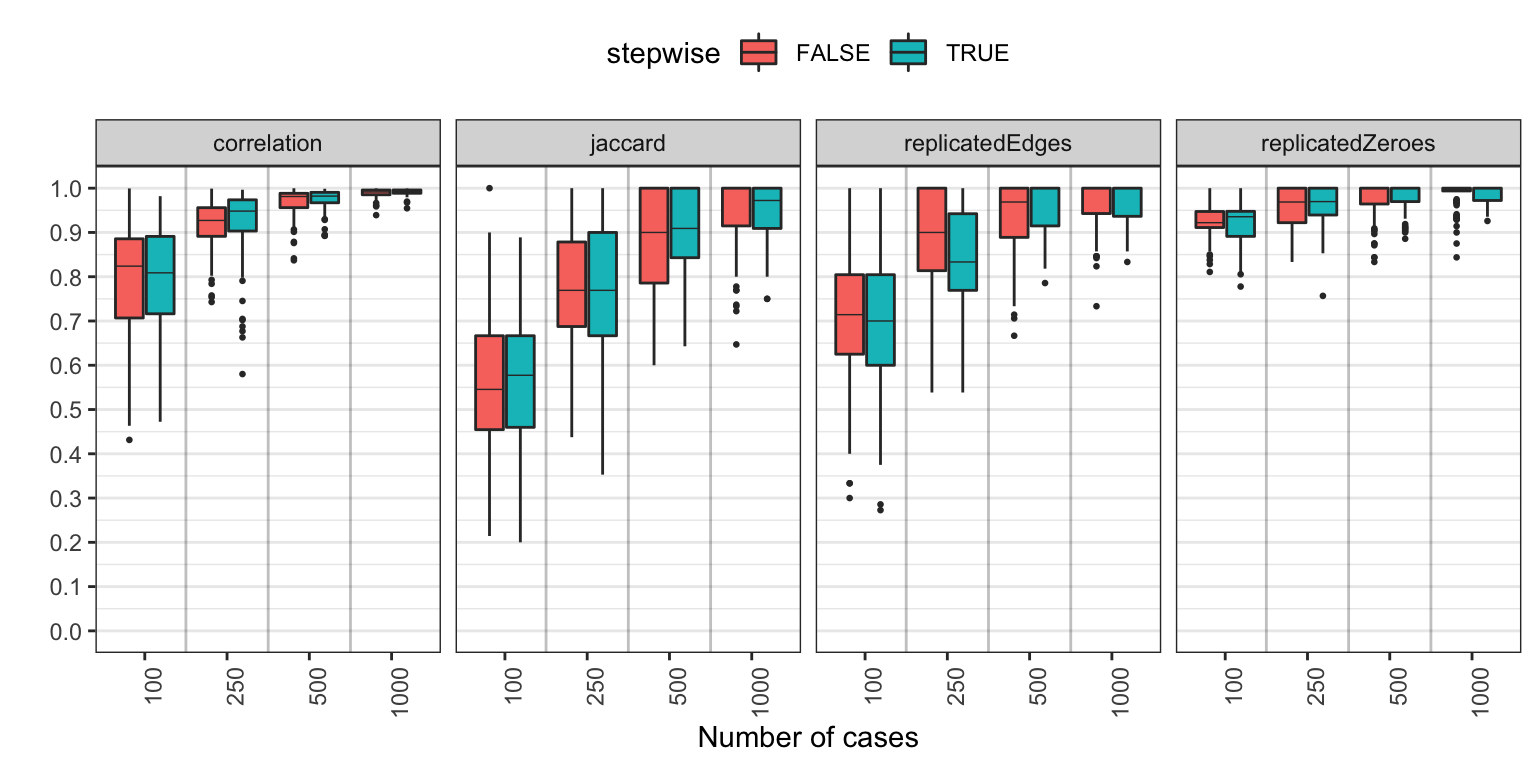# Future developments

As always, I highly welcome bug reports and code suggestions on Github. I would also welcome any volunteers willing to help work in this project. This work can include adding new default sets, but also overhauling the help pages or other work. Please contact me if you are interested!